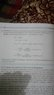# Solve Archimedes Problem: Find Crown's Density

• Dipra Irham
In summary, the conversation discusses a physics problem about the density of a gold crown and the confusion surrounding the use of weight and mass. The solution involves calculating the volume and density of the crown and using the weight of the crown in air and when immersed in water. There is also a discussion about the units used and the possible methods used by Archimedes to determine if the crown was made of pure gold.

#### Dipra Irham

I have got a question in my physics book.The question goes like that:
(Let's suppose the weight of the gold crown of Archimedes is 10 kg in air and 9.4 kg when immersed in water.What is the density of the crown? )
The solution says:
If the volume of the crown is V and density is p then,
Vp=10 kg
Vp-(Vp of water)=9.4 kg
Vp of water = Vp - 9.4 kg= 0.6 kg
so, V = 0.6 kg / density of water
=0.6 kg÷10^3 kg/m^3= 0.6 X 10^ -3m^3
Therefore, p = 10 kg / V
= 10 kg/0.6 X 10^ -3m^3
=16666 Kg /m^3
my question is, why Vp =10 kg?(it should be Vp times acceleration due to gravity,since weight = mass X acceleration due to gravity)

#### Attachments

•IMG_20180603_230146.jpg
27.6 KB · Views: 476
Dipra Irham said:
I have got a question in my physics book.The question goes like that:
(Let's suppose the weight of the gold crown of Archimedes is 10 kg in air and 9.4 kg when immersed in water.
Technically, the mass of the gold crown is 10 kg in air. The crown would have the same mass on the moon with its lower gravity, but its weight would be less. The proper unit of weight in this problem would be Nt (Newtons).

Immersing the crown in water wouldn't change its mass.
Dipra Irham said:
What is the density of the crown? )
The solution says:
If the volume of the crown is V and density is p then,
Vp=10 kg
Vp-(Vp of water)=9.4 kg
Vp of water = Vp - 9.4 kg= 0.6 kg
so, V = 0.6 kg / density of water
=0.6 kg÷10^3 kg/m^3= 0.6 X 10^ -3m^3
Therefore, p = 10 kg / V
= 10 kg/0.6 X 10^ -3m^3
=16666 Kg /m^3
my question is, why Vp =10 kg?(it should be Vp times acceleration due to gravity,since weight = mass X acceleration due to gravity)
The density here apparently is in units of ##\frac{\text{kg}}{m^3}##. Multiplying V and p results in units of kg. It's hard to tell what they're trying to say in this problem, as the units being used don't make sense.

BTW, the image you posted is too small to be readable, and part of it is in shadow, which makes it even less useful.

•Dipra Irham
The only error I see is in this opening statement: "weight ... is 10 kg in air and 9.4 kg when immersed in water."
That can be corrected either by including the factor g: "10g kg in air and 9.4g kg when immersed in water"
or by changing "kg" to "kg-weight".
Either way, the first equation becomes Vρg=10g kg, but the g's immediately cancel to give Vρ=10 kg.

•Dipra Irham
I would note that, in the time of Archimedes, Newtons were far in the future and so was the value of g. The "weighing" process was most likely conducted using an equal arms scale with an amount of gold equal to what was given to the craftsman in one pan and the crown in the other. In air they would balance, but with both pans immersed they would not if the crown were adulterated. In short it makes sense to imagine that Archimedes conducted what we now call a null measurement.

•Dipra Irham
haruspex said:
The only error I see is in this opening statement: "weight ... is 10 kg in air and 9.4 kg when immersed in water."
That can be corrected either by including the factor g: "10g kg in air and 9.4g kg when immersed in water"
or by changing "kg" to "kg-weight".
Either way, the first equation becomes Vρg=10g kg, but the g's immediately cancel to give Vρ=10 kg.